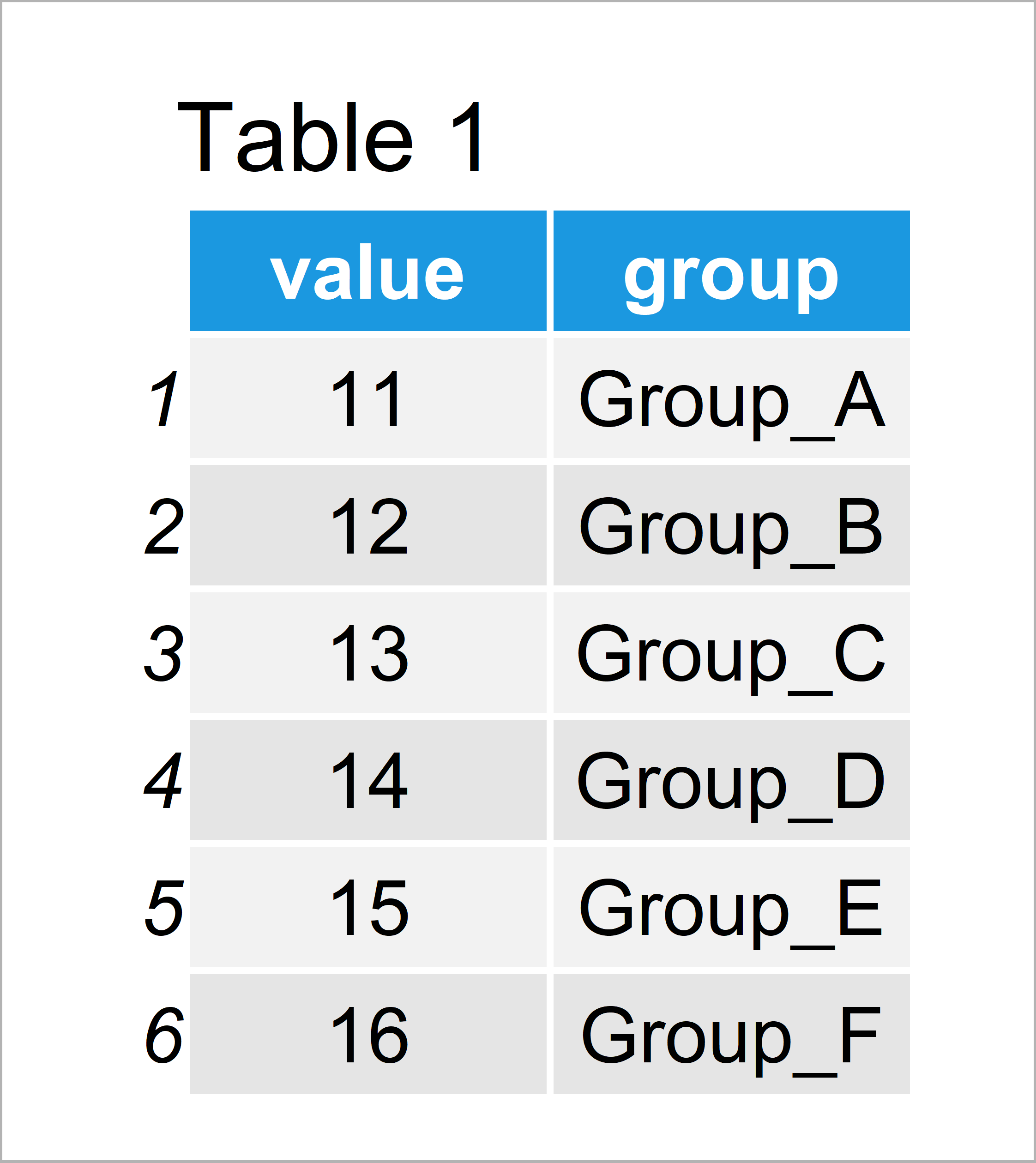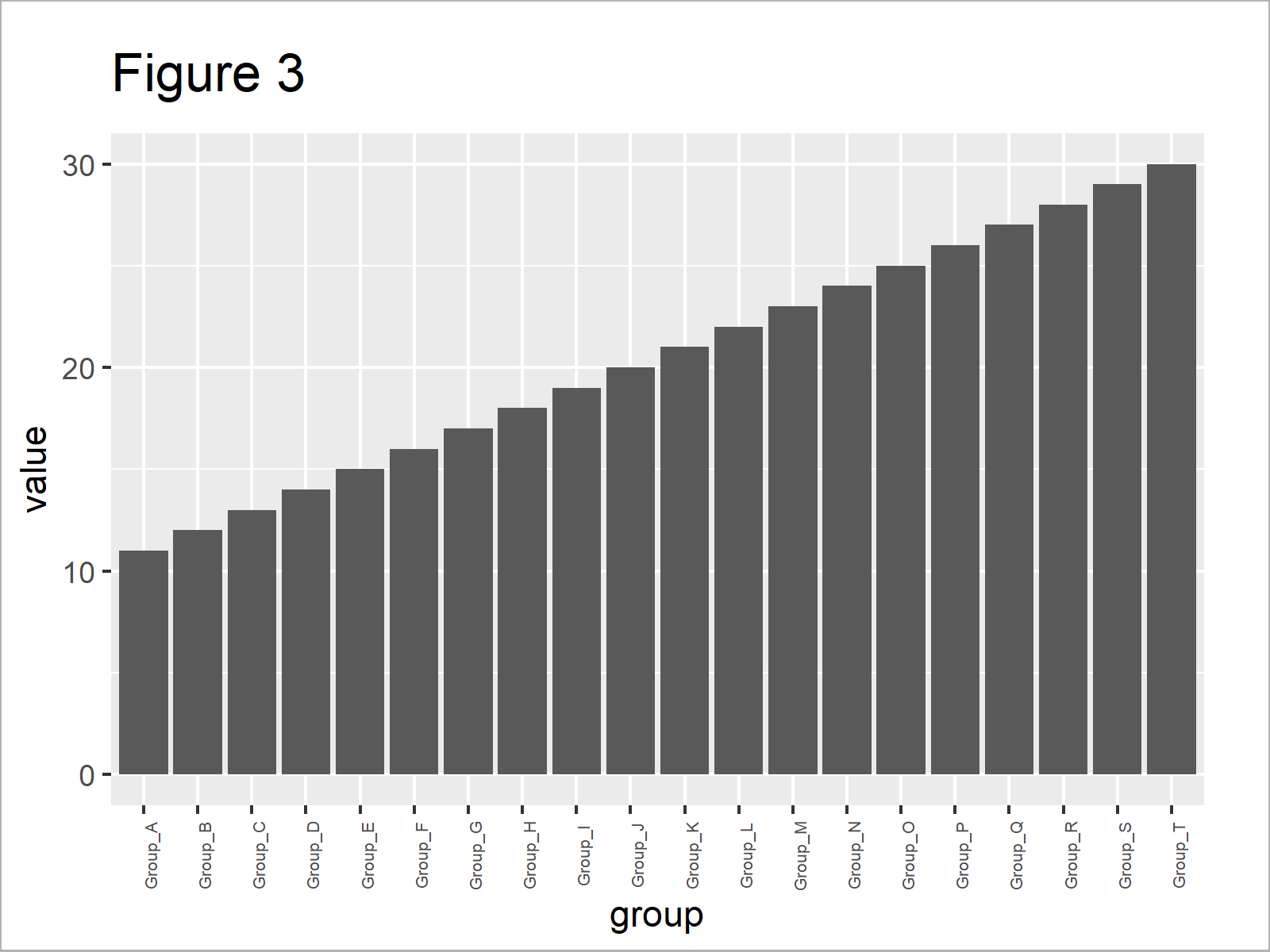# Display All X-Axis Labels of Barplot in R (2 Examples)

In this tutorial, I’ll show how to show every x-axis label of a barplot in R programming.

The article consists of these topics:

Let’s dive into it…

## Example Data & Default Graphic

We’ll use the data below as basement for this R programming tutorial:

```data <- data.frame(value = 11:30,    # Create example data
group = paste0("Group_", LETTERS[1:20]))Have a look at the table that got returned by the previous syntax. It shows that our example data has 20 rows and two columns. Each row represents a different bar of our barplot.

As next step, we can draw our data with default specifications:

`barplot(data\$value ~ data\$group)     # Default barchart in Base R`As shown in Figure 1, we have managed to create a bargraph by executing the previous code. However, since our graphic contains too many bars, not all axis labels are shown.

Let’s change that!

## Example 1: Show All Barchart Axis Labels of Base R Plot

Example 1 explains how to display all barchart labels in a Base R plot.

There are basically two major tricks, when we want to show all axis labels:

Let’s do both in R:

```barplot(data\$value ~ data\$group,     # Modify x-axis labels
las = 2,
cex.names = 0.7)```In Figure 2 you can see that we have created a barplot with 90-degree angle and a smaller font size of the axis labels. All text labels are shown.

## Example 2: Show All Barchart Axis Labels of ggplot2 Plot

In this example, I’ll show how to display all axis text labels of a ggplot2 graph.

In order to use the functions of the ggplot2 package, we first have to install and load ggplot2:

```install.packages("ggplot2")          # Install & load ggplot2 package
library("ggplot2")```

Next, we can use the theme function and the axis.text.x argument to change the angle and decrease the font size of the axis labels:

```ggplot(data, aes(group, value)) +    # ggplot2 plot with modified x-axis labels
geom_bar(stat = "identity") +
theme(axis.text.x = element_text(angle = 90, size = 5))```As shown in Figure 3, the previous syntax has drawn a ggplot2 barplot in which all axis labels are displayed.

## Video & Further Resources

Do you need more explanations on the R programming code of this tutorial? Then you may want to have a look at the following video of my YouTube channel. I illustrate the R code of this post in the video.

Please accept YouTube cookies to play this video. By accepting you will be accessing content from YouTube, a service provided by an external third party.If you accept this notice, your choice will be saved and the page will refresh.

Furthermore, you may want to have a look at the other tutorials on my homepage. A selection of articles about graphics in R is shown below.

To summarize: At this point you should know how to display all text labels of a barchart axis in the R programming language. Please note that we could use the same kind of syntax to show all labels of other types of graphics such as boxplots or heatmaps.

Subscribe to the Statistics Globe Newsletter

•Stephen
August 11, 2023 1:56 am

When I do the ggplot geom_bar, only a few X-axis labels show

•August 11, 2023 2:50 pm